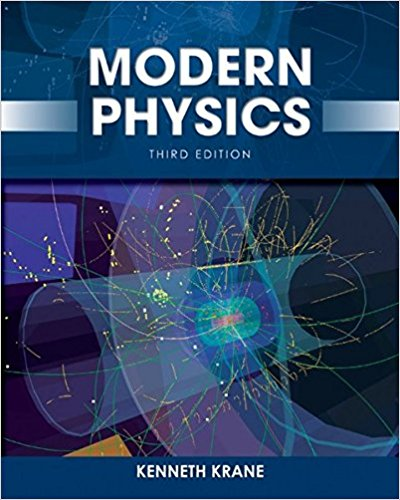Spring 2018

# home$$\beta = \frac{v}{c} \\ \gamma = \frac{1}{\sqrt{1 - \beta^2}} \\ ct' = \gamma ( ct - \beta x ) \\ x' = \gamma ( x - \beta ct ) \\$$

... and Quantum Mechanics.

$$\frac{\hbar^2}{2m}\nabla^2\Psi + V(\mathbf{r})\Psi = -i\hbar \frac{\partial\Psi}{\partial t}$$

Yes, you get both in one fun-filled semester!

https://cs.marlboro.college /cours /spring2018 /modern_physics /home